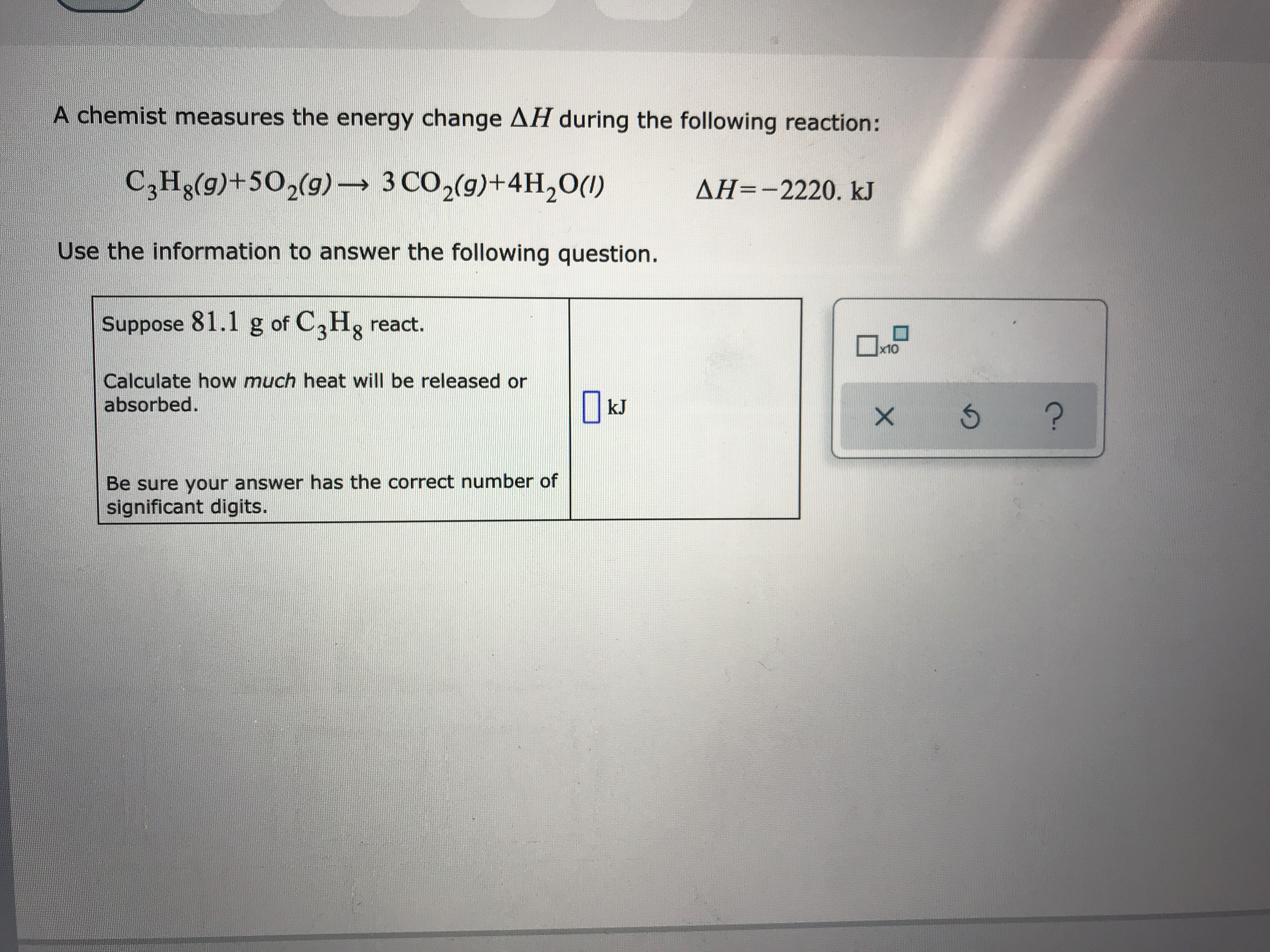# A chemist measures the energy change AH during the following reaction:C3Hg(g)+502(g) 3 CO2(g)+4H20()AH=-2220. kJUse the information to answer the following question.Suppose 81.1 g of C2Hg react.x10Calculate how much heat will be releasedabsorbed.kJ?Be sure your answer has the correct number ofsignificant digits.X

Question
10 viewshelp_outlineImage TranscriptioncloseA chemist measures the energy change AH during the following reaction: C3Hg(g)+502(g) 3 CO2(g)+4H20() AH=-2220. kJ Use the information to answer the following question. Suppose 81.1 g of C2Hg react. x10 Calculate how much heat will be released absorbed. kJ ? Be sure your answer has the correct number of significant digits. X fullscreen
check_circle

Step 1

The given reaction is shown below.

Step 2

The change in enthalpy for the given is -2220 kJ.

The negative sign of ∆H means that the reaction is an exothermic. It means that the system released a heat. Exothermic reactions are those reactions in which energy is released after the completion of reaction.

Step 3

The given weight of C3H8 is 81.1 g.

The chemical name of C3H8 is propane.

The molar mass of C3H8 is 44.1 g/mol.

The number of ...

### Want to see the full answer?

See Solution

#### Want to see this answer and more?

Solutions are written by subject experts who are available 24/7. Questions are typically answered within 1 hour.*

See Solution
*Response times may vary by subject and question.
Tagged in

### Chemistry